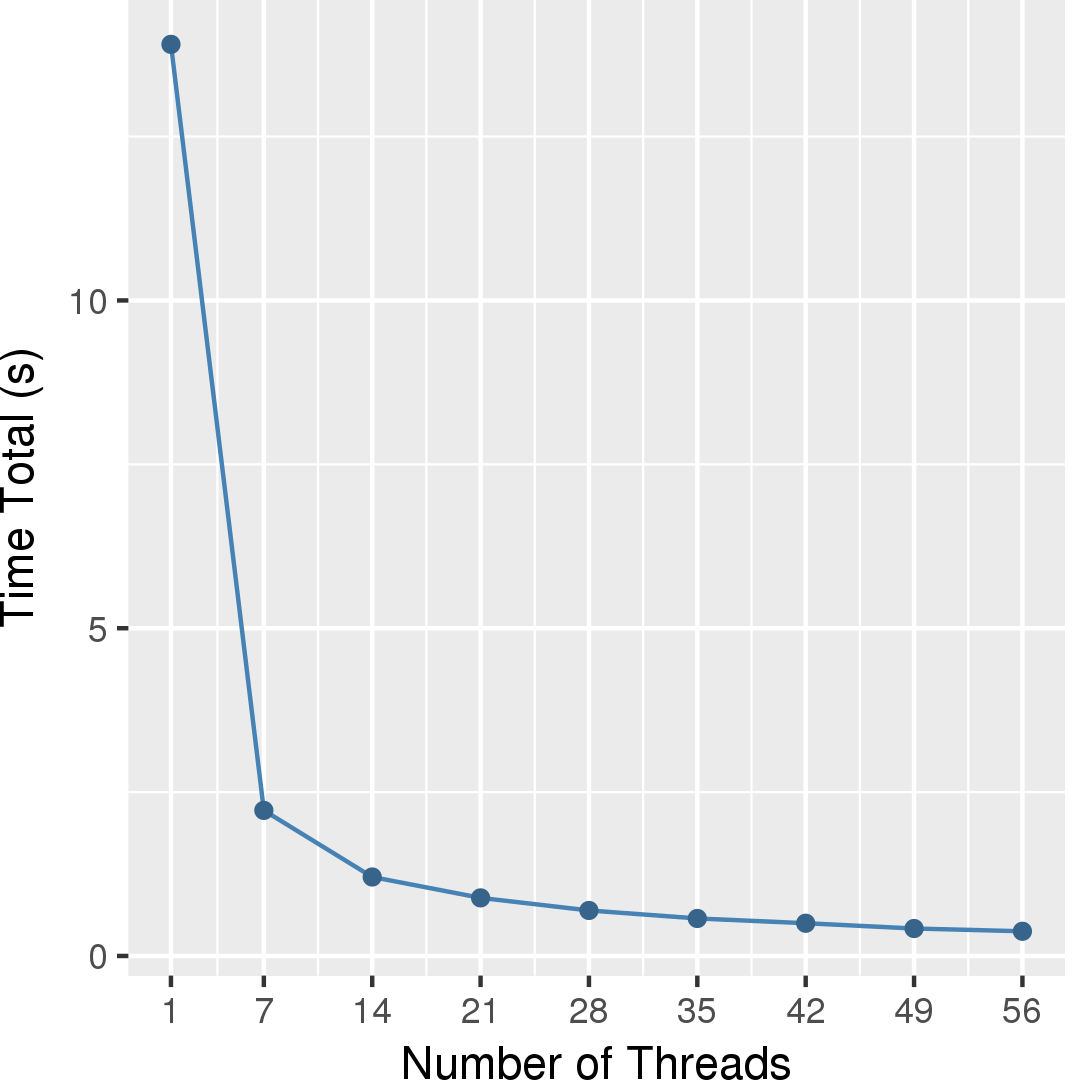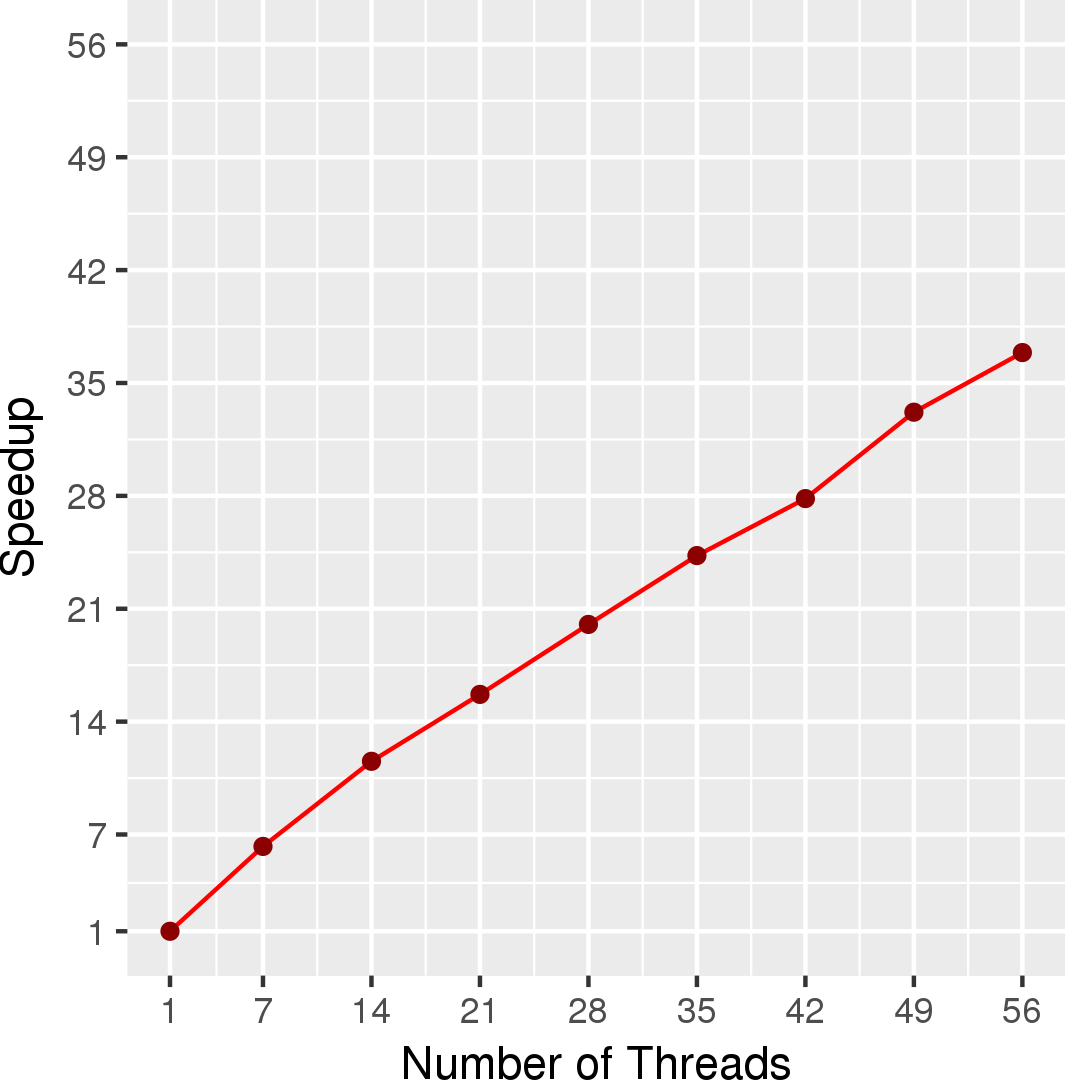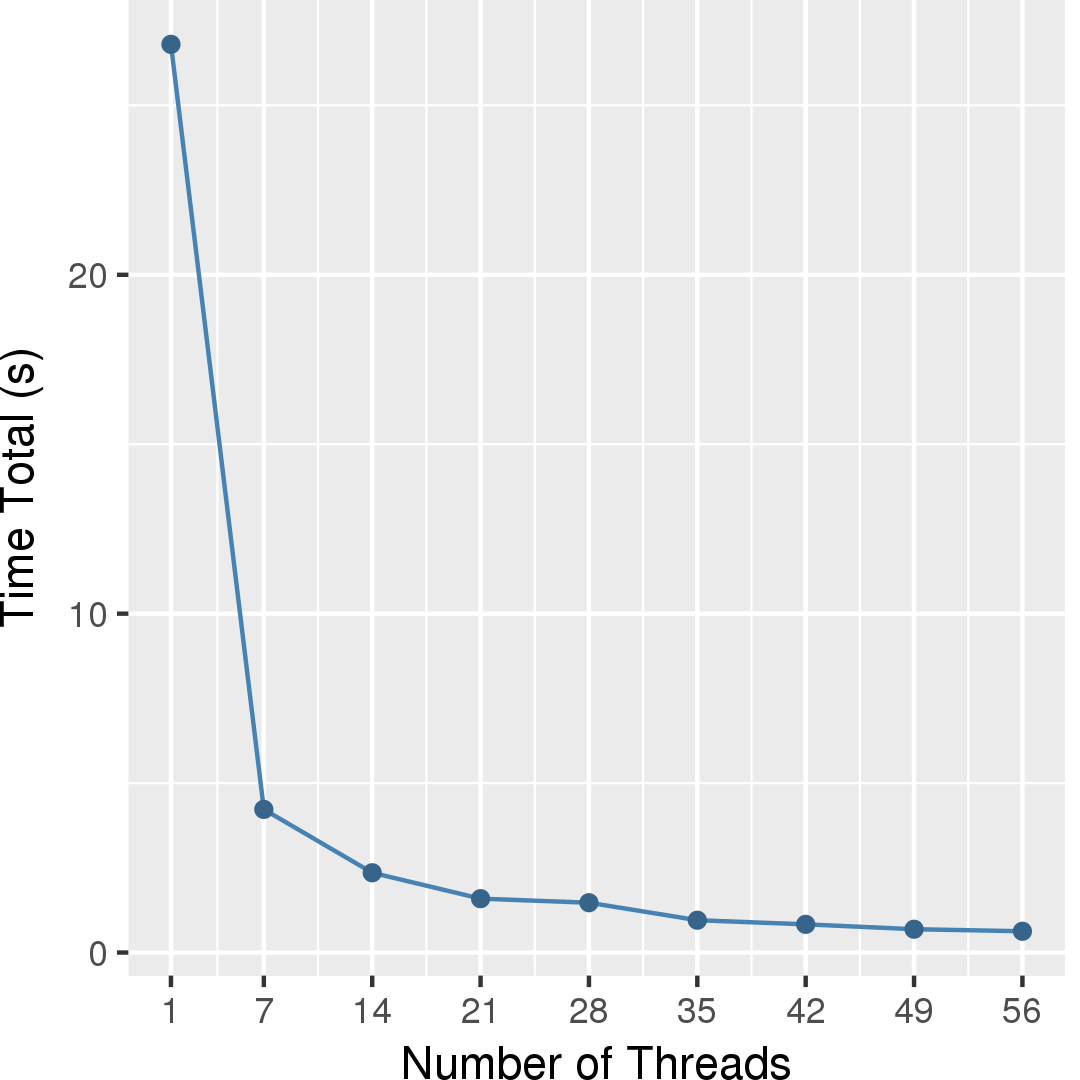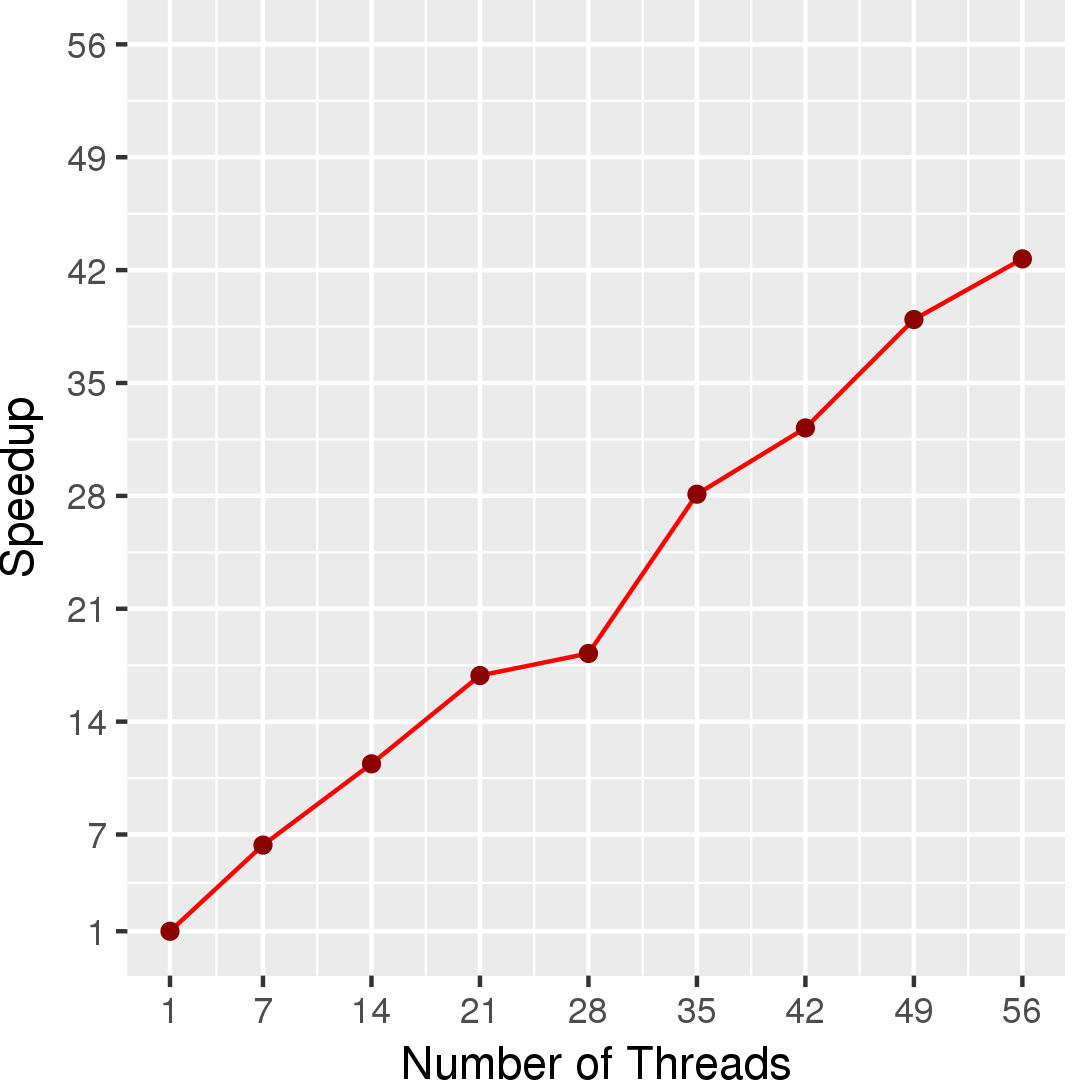# Triangle Counting

## Application Description

This benchmark counts the number of triangles in a given undirected graph. It implements both the node-iterator and edge-iterator algorithms in . All nodes/edges can be processed independently.

 Thomas Schank. Algorithmic Aspects of Triangle-Based Network Analysis. PhD Thesis. Universitat Karlsruhe. 2007.

## Algorithms

Node-iterator algorithm works as the following. For a pair of nodes a and b, both are node n's neighbors, if a is b's neighbor, and vice versa, then there is a triangle composed of nodes n, a, and b. Therefore, we can count triangles by scanning through all pairs of neighbors for all nodes and see if the pair of nodes are each other's neighbor. To avoid double counting, we count a triangle only if a < n < b by some criteria. This requires pre-sorting of edge lists for all nodes. Figure 1 shows the pseudocode for node-iterator algorithm.

 1 2 3 4 5 6 7 8 9 10 11 12 /* sort edge lists for all nodes */ num_triangles = 0; foreach (Node n: graph) { foreach (Node a: n's neighbor) { if (a >= n) continue; foreach (Node b: n's neighbor) { if (b > n and b is a's neighbor) { num_triangles++; } } } }

Figure 1: Pseudocode for node iterator algorithm of triangle counting

Edge-iterator algorithm works as the following. Given an edge e whose end points are n and m, if n and m have a common neighbor a, then there is a triangle formed by nodes n, m, and a. Hence, we can count triangles by intersecting each edge's end points' edge lists and summing all intersections' sizes. To avoid double counting, we only consider n < a < m by some criteria when intersecting edge lists. This requires pre-sorting of edge lists for all nodes. Figure 2 shows the pseudocode for edge-iterator algorithm.

 1 2 3 4 5 6 7 8 9 /* sort edge lists for all nodes */ num_triangles = 0; foreach (Edge e: graph) { Node n = e.src; Node m = e.dst; NodeSet n_ngh = {s| s is n's neighbor and n < s < m}; NodeSet m_ngh = {t| t is m's neighbor and n < t < m}; num_triangles += |n_ngh intersect m_mgh|; }

Figure 2: Pseudocode for edge-iterator algorithm of triangle counting

## Data Structures

There input graph is the data structure used in Triangle Counting for both node-iterator and edge-iterator algorithm.

## Parallelism

Both node-iterator and edge-iterator algorithms are topology-driven algorithms, since all nodes/edges are active. The graph is only read, so all activities at nodes/edges can proceed independently.

## Performance

Figures 2 and 4 show the execution time of the edge-iterator algorithm and of the node-iterator algorithm, respectively. Figures 3 and 5 show the self-relative speedup, i.e, speedup with respect to the single thread performance for edge-iterator algorithm an node-iterator algorithm, respectively. The input is LiveJournal graph for both algorithms.## Machine Description

Performance numbers are collected on a 4 package (14 cores per package) Intel(R) Xeon(R) Gold 5120 CPU machine at 2.20GHz from 1 thread to 56 threads in increments of 7 threads. The machine has 192GB of RAM. The operating system is CentOS Linux release 7.5.1804. All runs of the Galois benchmarks used gcc/g++ 7.2 to compile.